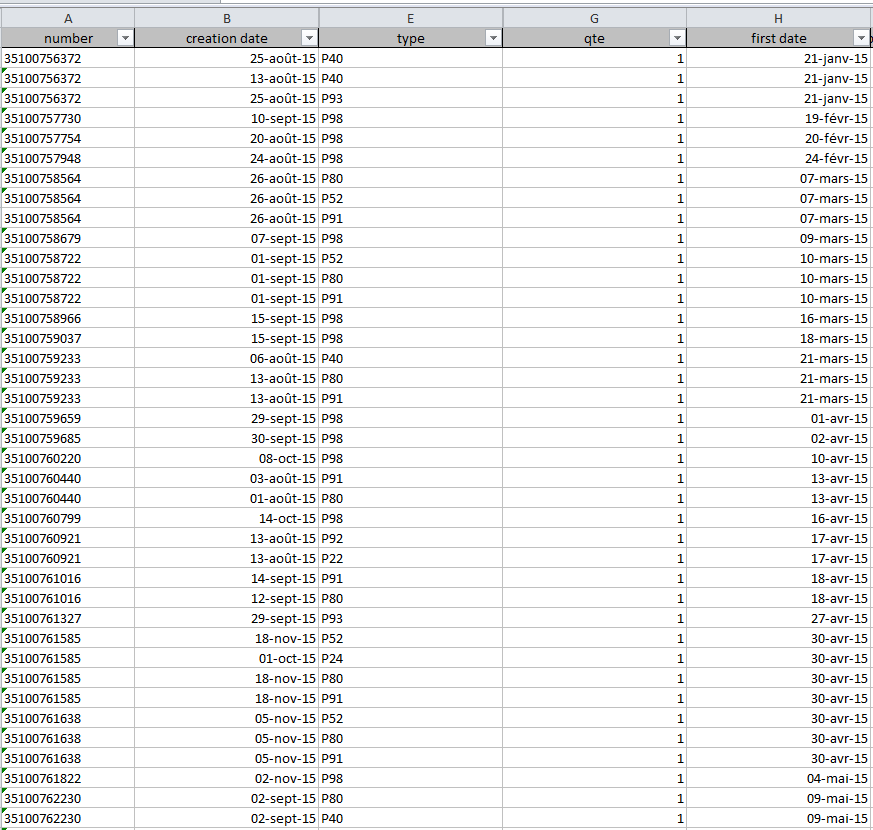# New to QlikView

Discussion board where members can get started with QlikView.

Announcements
Save the Date: QlikWorld Online, June 24-25, 2020. Free global virtual event for data integration and data analytic gurus. Register TodayNot applicable

## Calculate delay from a single row

Hello,

I have to determine the delay between 2 type, but these date are in the same row named creation date.

For example I have to know the delay between P40 and P91 or above. For this I use the NetWorkDays function and it work well when I'm working with the delay from the first date to the creation date. I wrote this :

Avg(NetWorkDays([first_date],if(type='P91' or type='P92'or type='P93'or type='P98',[creation date])))

Here I want the average delay from the first date to the finalisation of a contract which correpond to P91 and above from the creation date. And it's work fine

the problem is when I only use the creation date to determine the delay between P40 and P91 and above it doesn't work because I'm working in the same row.

Avg(NetWorkDays(if(type='P40',[creation date]),if(type='P91' or type='P92'or type='P93'or type='P98',[creation date])))

This display any data. If someone have some idea how it can work, that would be greatThank you1 Solution

Accepted Solutions
HighlightedMVP

## Re: Calculate delay from a single row

Maybe something like

=Avg(

Aggr(

NetWorkDays( Max({<type={'P40'}>} [creation date]) , Min( {<type ={'P91','P92','P93','P98'}>} [creation date]) )

,number)

)

So, for each number, look for the max P40 creation date and calculate networkdays to min creation date with either {'P91','P92','P93','P98'}.

Then calculate the avg number of networkdays delay across numbers.

Hope this helps,

Stefan

3 Replies
HighlightedMVP

## Re: Calculate delay from a single row

It would be much easier to help if you can provide a sample. Looking at an image and trying to decipher the expression is quite challenging.

Best,

Sunny

HighlightedMVP

## Re: Calculate delay from a single row

Maybe something like

=Avg(

Aggr(

NetWorkDays( Max({<type={'P40'}>} [creation date]) , Min( {<type ={'P91','P92','P93','P98'}>} [creation date]) )

,number)

)

So, for each number, look for the max P40 creation date and calculate networkdays to min creation date with either {'P91','P92','P93','P98'}.

Then calculate the avg number of networkdays delay across numbers.

Hope this helps,

Stefan

HighlightedNot applicable

## Re: Calculate delay from a single row

Thank you !It worked fine, the aggr function was the answer